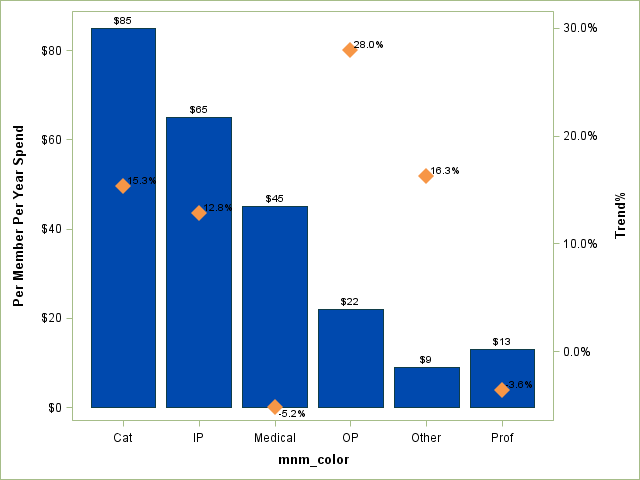## SAS Graphs (Annotation + Proc Gbarline)

Hi All,

how can i annotate the values correctly if i have +ve & -ve values in data. My code works well for most, if the value is +ve. If the values are negative then output is disorientated. the annotation values dont tie up with  diamond symbol on graph. below is the code, how should i automate if my values are either +ve or -ve .  here is the code.

%let name = test;
PROC FORMAT library=work;
picture pct0dec (round) low -< 0 = '00009%' (prefix='-')
0 - high = '00009%' (prefix='');

picture pct1dec (round) low -< 0 = '00009.9%' (prefix='-')
0 - high = '00009.9%' (prefix='');

picture pct2dec (round) low -< 0 = '00009.99%' (prefix='-')
0 - high = '00009.99%' (prefix='');

RUN;

data my_data1;
length mnm_color \$10;
format count dollar10. value pct1dec.;
input mnm_color count value;
datalines;
Medical 45 -5.2
Cat 85 15.3
IP 65 12.8
OP 22 28.0
Prof 13 -3.6
Other 9 16.3
;
run;

proc sql noprint;
select max(int(value)) into: max_val from work.my_data1;
quit;
%put &max_val.;

data myanno_1; set my_data1;
length function color \$8;
xsys='2'; when='a'; function='label';
midpoint=mnm_color;
ysys='1';
y=0; text=trim(left(put(count,dollar10.)));
color='green'; position='2';size=2;style='Regular'; output;
if value > 0 then do;
y=((value-1.0)/(30-1.0))*100 ;
text=trim(left(put(value,pct1dec.)));
color='white'; position='1'; size=2;style='Regular';CBOX='green';output;
end;
else if value = 0 then do;
y=(&max_val.+4)*2;
text=trim(left(put(value,pct1dec.)));
color='white'; position='1'; size=2;style='Regular';CBOX='green';output;
end;
else do;
y = (&max_val.+4)*2;
text=trim(left(put(value,pct1dec.)));
color='white'; position='6'; size=2;style='Regular';CBOX='green';output;
end;

run;

/*options linesize=MAX pagesize=MAX;*/
goptions reset=all noborder cback=white htitle=12pt htext=10pt imagestyle= vsize=5in;
goptions xpixels=525 ypixels=400;
goptions border;

ODS LISTING CLOSE;
ODS HTML path='physicalpath' body="&name..htm" style=htmlblue
gpath='physicalpath/Test.jpg';

goptions ftitle="albany amt/bold" ftext="albany amt/bold"
gunit=pct ;
/*htitle=5.0 htext=3.2 ctext=gray33;*/

axis1 value=(angle=360 rotate=0) label=none ;
axis2 label=(angle=90 "Per Member Per Year Spend") value=none ;/*order=(0 to 1100000 by 100000);*/
axis3 label=(angle=90 "Trend%") label=none value=none order=(-32 to 32 by 2); /*order=(-20 to 20 by 2)*/
symbol1 interpol=none value=diamondfilled color='#F79646' height=6 ;
pattern1 v=solid color='#0049AE';

proc gbarline data= my_data1;
/*symbol font= value=diamondfilled co=mob HEIGHT=5 interpol=none ;*/
bar mnm_color / sumvar=count maxis=axis1 raxis=axis2 NOFRAME Noheading
midpoints="Medical" "Cat" "IP" "OP" "Prof" "Other" width=16 noaxis anno=myanno_1 ; /*anno=myanno*/
plot / sumvar=value raxis=axis3 ;
run;
quit;

ODS HTML CLOSE;
ODS LISTING;

1 ACCEPTED SOLUTION

Accepted Solutions

## Re: SAS Graphs (Annotation Proc Gbarline)I didn't use many options.

9 REPLIES 9

## Re: SAS Graphs (Annotation + Proc Gbarline)

Without data to plot (the actual data not the annotate) or a picture of the result it isn't quite clear what your issue is.

I do know that I would strongly suggest looking at Proc SGPlot with Vbar and Vline statements as Gbarline is pretty limited to the main display.

## Re: SAS Graphs (Annotation + Proc Gbarline)

data my_data1; length mnm_color \$10; format count dollar10. value pct1dec.; input mnm_color count value; datalines; Medical 45 -5.2 Cat 85 15.3 IP 65 12.8 OP 22 28.0 Prof 13 -3.6 Other 9 16.3 ; run;

this is the sample data i am using.

## Re: SAS Graphs (Annotation + Proc Gbarline)

@pmksasbi wrote:

data my_data1; length mnm_color \$10; format count dollar10. value pct1dec.; input mnm_color count value; datalines; Medical 45 -5.2 Cat 85 15.3 IP 65 12.8 OP 22 28.0 Prof 13 -3.6 Other 9 16.3 ; run;

this is the sample data i am using.

And you have a good example why code and log results should be pasted into a code box using the forum {i} icon.

## Re: SAS Graphs (Annotation + Proc Gbarline)

I get an error from this line:

symbol1 interpol=none value=diamondfilled color='#F79646' height=6 ;

At least in my install diamondfilled is not acceptable for SYBMOL statement value and generates an error.

Thanks

attachments. -##

## Re: SAS Graphs (Annotation Proc Gbarline)

I'm not sure about your calculations for the y offset for the annotation.

I know the below doesn't fit all of your need but you might consider this as a different starting point.

```proc sgplot data=work.my_data1 noautolegend ;
vbar mnm_color /response=count datalabel Fillattrs=(color='cx0049AE');
vline mnm_color/response=value y2axis datalabel name='vline'
markers markerattrs=(color='cxF79646' size=10pt symbol=diamondfilled)
lineattrs=(thickness=0)
;
yaxis label="Per Member Per Year Spend" ;
y2axis label= 'Trend%' ;
run; ```

There are additional text options, axis options and attrmap dataset that may help.

## Re: SAS Graphs (Annotation Proc Gbarline)

Thanks for the reply. I have Javav error's. i will have to check with my system admin. If possible can you please attach the output. i am just curious to see how the output looks. Thanks

ERROR: The Java proxy is not responding.
ERROR: Unable to load the Java Virtual Machine. Please see the installation
ERROR: Unable to load the Java Virtual Machine. Please see the installation
ERROR: The Java child process terminated unexpectedly: status 255.
ERROR: WIFSIGNALED 0 WIFEXITED 0 WEXITSTATUS -1 WTERMSIG -1.
NOTE: The SAS System stopped processing this step because of errors.
NOTE: There were 6 observations read from the data set WORK.MY_DATA1.
NOTE: Remote submit to ATLAS complete.

## Re: SAS Graphs (Annotation Proc Gbarline)I didn't use many options.

## Re: SAS Graphs (Annotation Proc Gbarline)

Thanks

Discussion stats
• 9 replies
• 1717 views
• 1 like
• 2 in conversation